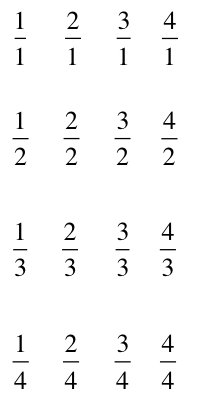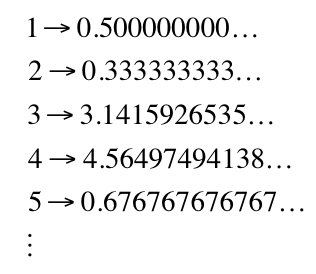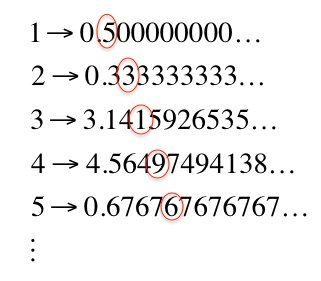Infinity is a powerful concept. Philosophers, artists, theologians, scientists, and people from all walks of life have struggled with ideas of the infinite and the eternal throughout history.

Infinity is also an extremely important concept in mathematics. Infinity shows up almost immediately in dealing with infinitely large sets – collections of numbers that go on forever, like the natural, or counting numbers: 1, 2, 3, 4, 5, and so on.

Infinite sets are not all created equal, however. There are actually many different sizes or levels of infinity; some infinite sets are vastly larger than other infinite sets.

The theory of infinite sets was developed in the late 19th century by the brilliant mathematician Georg Cantor. Many of Cantor’s ideas and theorems sit at the foundation of modern mathematics. One of Cantor’s coolest innovations was a way to compare the sizes of infinite sets, and to use this idea to show that there are many infinities.

To see how Cantor’s theory works, we start out by saying that two sets are the same size if we can make a one to one correspondence, or pairing up, of the elements of the two sets. We can start small – the sets {a, b, c} and {1, 2, 3} are the same size, since I can pair up their elements:This is a little overcomplicated for comparing two small finite sets like these – it is obvious that they both have three elements, and so are the same size. However, when we are looking at infinite sets, we cannot just look at the sets and count up the numbers of elements, since the sets go on forever. So, this more formal definition will be very helpful.

Countably Infinite Sets

Our baseline level of infinity will come from our most basic infinite set: the previously mentioned natural numbers. A set that is the same size as the natural numbers – that can be put into a one to one correspondence with the natural numbers – is called a countably infinite set.

A surprising number of infinite sets are actually countable. At first glance, the set of integers, made up of the natural numbers, their negative number counterparts, and zero, looks like it should be bigger than the naturals. After all, for each of our natural numbers, like 2 or 10, we just added a negative number, -2 or -10. But the integers are countable – we can find a way to assign exactly one integer to each natural number by bouncing back and forth between positive and negative numbers:If we continue the pattern suggested above, we end up assigning exactly one integer to each natural number, with each integer assigned to a natural number, giving us the kind of one to one pairing that means the two sets are the same size.

This is a little freaky, since the natural numbers are a subset of the integers – each natural number is also an integer. But even though the natural numbers are fully contained in the integers, the two sets actually do have the same size.

The rational numbers are those numbers that can be written as a fraction, or ratio, of two integers: 1/2, -5/4, 3 (which can be written as 3/1), and the like. This is another infinite set that looks like it should be bigger than the natural numbers – between any two natural numbers, we have infinitely many fractions.

But as with the integers, we can still make a one to one pairing, assigning exactly one natural number to each rational number. Start by making a grid of the rationals: each row has a particular natural number in the bottom part of the fraction – the denominators of the first row are all 1’s, and the 2nd row all 2’s. Each column has a particular number in the top part of the fraction – the numerators of the first column are all 1’s, and the second column all 2’s. This grid covers all of the positive rational numbers, since any ratio of two positive integers will show up somewhere in the grid:We get our correspondence between the rationals and the naturals by moving in a zig-zag pattern through the grid and counting. Fractions like 2/2 and 4/6 that are just alternate representations of numbers we have already seen (2/2 is the same as 1/1, and 4/6 is the same as 2/3) are skipped over:So, the first rational number is 1/1, the second is 2/1, the third is 1/2, the fourth is 1/3, we skip 2/2 since this just reduces to 1/1, the fifth is 3/1, and so on.

Continuing like this, every rational number will be assigned a unique natural number, showing that, like the integers, the rationals are also a countably infinite set.

Even though we have added all these fractions and negative numbers to our original basic natural number set, we are still at our first, baseline, level of infinity.

Uncountably Infinite Sets

Now we consider the real numbers. The real numbers are the collection of numbers that can be written out with some kind of decimal expansion. The real numbers include the rational numbers – any fraction of two integers can be divided out and turned into a decimal. 1/2 = 0.5 and 1/3 = 0.3333…, with the latter continuing on with 3’s forever. The real numbers also include irrational numbers, or decimals that go on forever without settling into a repeated pattern or ending. π is irrational – its decimal expansion starts out with the familiar 3.14159… but keeps going on forever, its digits veering around wildly.

We were able to come up with clever correspondences with the natural numbers for the integers and the rationals, showing that they are all countably infinite and the same size. Given that, we might think that we can do something similar with the real numbers.

This is, however, impossible. The real numbers are an uncountably infinite set – there actually are far more real numbers than there are natural numbers, and there is no way to line up the reals and the naturals so that we are assigning exactly one real number to each natural number.

To see this, we use an extremely powerful technique in mathematics: proof by contradiction. We will start out by hypothesising that the opposite of our claim is true – that the real numbers are countably infinite, and so there is a way to line up all the reals with the naturals in a one to one correspondence. We will see that it doesn’t matter exactly what this correspondence looks like, so let’s say that the first few pairs in the correspondence are the following:Our big assumption here is that each and every real number appears somewhere on this list. We are now going to show that this is in fact wrong by making a new number that does not show up in the list.

For each natural number n, we look at the corresponding real number on the list, and take the digit n places to the right of the real number’s decimal point. So, take the first digit of the first number, the second digit of the second number, the third digit of the third number, and so on:From our first real number we get a 5, our second number a 3, and our third number a 1. We make a new number by taking each of these digits, and adding 1 to them (flipping around to a 0 if my original digit is 9), giving us the number 0.64207…, continuing on for all the other numbers on our list.

This new “diagonal” number is definitely a real number – it has a decimal expansion. But it is different from all the numbers on the list: its first digit is different from the first digit of our first number, its second digit is different from the second digit of our second number, and so on.

We have made a new real number that does not show up on our list. This contradicts our main assumption that every real number appears somewhere in the correspondence.

So…

We mentioned before that the details of the correspondence did not matter. This is because, no matter what alignment we try between the real numbers and the natural numbers, we can do the same diagonal trick above, making a number that does not show up in the correspondence.

This shows that the reals are not countably infinite. No matter what we try, there is no way to make a one to one pairing up of the natural numbers and the real numbers. These two sets are not the same size. This leads to the profound and somewhat uncomfortable realisation that there must be multiple levels of infinity – the natural numbers and the real numbers are both infinite sets, but the reals form a set that is vastly larger than the naturals – they represent some ‘higher level’ of infinity.

This site uses Akismet to reduce spam. Learn how your comment data is processed.